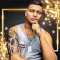Class Central is learner-supported. When you buy through links on our site, we may earn an affiliate commission.

### Overview

CS 224 is an advanced course in algorithm design, and topics we will cover include the word RAM model, data structures, amortization, online algorithms, linear programming, semidefinite programming, approximation algorithms, hashing, randomized algorithms, fast exponential time algorithms, graph algorithms, and computational geometry.

### Syllabus

Advanced Algorithms (COMPSCI 224), Lecture 1.
Advanced Algorithms (COMPSCI 224), Lecture 2.
Advanced Algorithms (COMPSCI 224), Lecture 3.
Advanced Algorithms (COMPSCI 224), Lecture 4.
Advanced Algorithms (COMPSCI 224), Lecture 5.
Advanced Algorithms (COMPSCI 224), Lecture 6.
Advanced Algorithms (COMPSCI 224), Lecture 7.
Advanced Algorithms (COMPSCI 224), Lecture 8.
Advanced Algorithms (COMPSCI 224), Lecture 9.
Advanced Algorithms (COMPSCI 224), Lecture 10.
Advanced Algorithms (COMPSCI 224), Lecture 11.
Advanced Algorithms (COMPSCI 224), Lecture 12.
Advanced Algorithms (COMPSCI 224), Lecture 13.
Advanced Algorithms (COMPSCI 224), Lecture 15.
Advanced Algorithms (COMPSCI 224), Lecture 16.
Advanced Algorithms (COMPSCI 224), Lecture 17.
Advanced Algorithms (COMPSCI 224), Lecture 18.
Advanced Algorithms (COMPSCI 224), Lecture 19.
Advanced Algorithms (COMPSCI 224), Lecture 20.
Advanced Algorithms (COMPSCI 224), Lecture 21.
Advanced Algorithms (COMPSCI 224), Lecture 22.
Advanced Algorithms (COMPSCI 224), Lecture 23.
Advanced Algorithms (COMPSCI 224), Lecture 24.
Advanced Algorithms (COMPSCI 224), Lecture 25.
Advanced Algorithms (COMPSCI 224), Lecture 26.

### Taught by

Harvard University

## Reviews

5.0 rating, based on 2 Class Central reviews

•